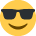# Pev help!!!

Hiya there!
I am extremely stuck on a question from my student kaplan notes - i have now been looking at it for 3 days!!!
Its quite long but here goes...

The standard cost of a sweater...
Direct Material: Quantity: 3kgs Unit Price: £4 Total Cost: £12
Direct Labour: Quantity: 1hr Unit Price: £6 Total Cost: £6
Fixed O/H Prod: Quantity: 1hr Unit Price: £2 Total Cost: £2
Total Standard Cost: £20

Actual material purchased: 25000kg
Actual cost of purchases £110000
Actual production: 6500 sweaters
Actual material used 20800kg
Direct labour hours actually worked 7150
Labour rate variance: 1430 (A)
Budgeted Labour hours: 7500
There was no change in stock of the sweater held during the month

Calculate:
1a) The standard usage of material
b) The standard labour time
c) The actual price paid per kg

2a) The material price varianceThe material usage variance
c) The labour efficiency variance
d) The total cost of labour used
e) The fixed overhead expenditure variance
f) The fixed overhehad volume variance
g) The fixed overhead efficiency variance
h) The fixed overhead capacity variance

I am fine upto 2d, then i dont understand where i get my budged production and my budgeted overhead cost from!
any help would be much appreciated so i can get this thing to reconcile!

Thanks for your time - hope your all having a good weekend!!!!!!

• My guess would be that as you are given the budgeted labour hours, you would calculate the budgeted production and overhead costs using the standard cost information.

i.e if the standard labour time is 1hr per sweater, and you are budgeted for 7,500 labour hours, your budgeted output will be 7,500 sweaters. To calculate the budgeted overhead, the standard cost is £2 per sweater, therefore £2 x 7,500 sweaters = £15,000

I'm not 100% sure that this is correct as costing was not my strongest subject!!
• I agree with Richard
If you want to compare my answer with yours, email me.
Sandy
[email protected]
www.sandyhood.com
sandy i have sent an email to the address below your name and i have recieved an auto message out of office reply - is this the right email address??

thankyou!
• Gemma

Per my reply to your message, I'm not certain that these answers are correct - especially the ones you are interested in RE the overhead variances bur here goes (starting with the answers to 1A):

Standard Material Usage (Based on actual production) = 6,500*3kg = 19,500kg
Standard Labour Time (actual production) = 6,500 * 1 = 6,500hrs
Price per kg = 110,000/25,000 = £4.40

Does this agree with what you have so far?

If so, moving on to question 2:

Material Price Variance = 8,320A (20,800*(4.40-4.00)) – kgs used (not including stock) multiplied by the difference between actual and budgeted cost

Material Usage Variance = 5,200A ((20,800-19,500)*4.00) – kgs used minus standard kgs that should’ve been used multiplied by standard cost

Labour Efficiency Variance = 3,900A ((7,150-6,500)*6.00) – difference between actual hours used and standard hours needed for 6500 units multiplied by labour cost

Cost of labour = £44,330 ((7,150*6.00) + 1,430) – the actual hours used at the standard rate plus the adverse variance of 1,430 already calculated in the question. (This equates to an actual cost per hour of £6.20 and 0.20*7,150 = 1,430)

Have we got the same answers up to this point? As of now I’m not so sure of my figures but here goes:

Fixed overhead expenditure variance = 850A (15,850 – (7,500*2.00)) – at an OAR of £2 per the question and a budget of 7,500 hours, you would expect to absorb £15,000 of overheads. You actually spent £15,850, hence the overhead expenditure variance.

Fixed overhead volume variance = 2,000A ((7,500-6500)*2.00) – you planned to do 7,500 hours and the standard hours needed for 6,500 units is 6,500hrs.

Fixed overhead capacity variance = 700A ((7,500-7,150)*2.00) – the difference between full capacity and what you actually used. You could’ve worked another 350 hours but didn’t, therefore an adverse variance.

Fixed overhead efficiency variance = 1300A ((7,150-6500)*2.00) – the difference between the actual hours and the hours you should’ve taken to produce 6,500 units.

These figures can be reconciled but as mentioned, I am not convinced of their accuracy so it could be that I’ve picked the wrong figures to balance to. My reconciliation, rightly or wrongly, is shown below:

Actual cost of actual production: £151,700 ((20,800*4.40)) + 44,330 + 15,850

8,320A MPV
5,200A MUV
1,430A LRV (Given)
3,900A LEV
850A FOEV
2,000A FOVV

21,700A total variances

151,700 – 21,700 = £130,000 Standard cost of actual production ((6,500*3*4.00) + (6,500*1*6.00) + (6,500*1*2.00))

If someone else could confirm my workings or correct my mistakes if I've gone about this in the wrong way, I would be very grateful.:thumbup1:
• I have calculated and reconciled the variances, and I agree with all of the answers that Adam has provided.
• I agree with all except the material price variance.

On revision days whenever we have to deal with questions where the stock of raw materials has changed due to purchased and used materials differing, this tends to cause problems.

Remember what the material price variance is intended to show management, so take the increase of 4,200kgs out of the variances and report that separately (it is not a variance).

Sandy
Sandy
[email protected]
www.sandyhood.com
• I thought I'd removed the stock from my calculation?

Thanks for confirming the rest though!:thumbup1: